# 十六、朴素贝叶斯

## 伯努利朴素贝叶斯

``````# 加载库
import numpy as np
from sklearn.naive_bayes import BernoulliNB

# 创建三个二元特征
X = np.random.randint(2, size=(100, 3))

# 创建二元目标向量
y = np.random.randint(2, size=(100, 1)).ravel()

# 查看前十个观测
X[0:10]

'''
array([[1, 1, 1],
[0, 1, 0],
[1, 1, 1],
[0, 0, 0],
[1, 0, 1],
[1, 1, 1],
[0, 1, 1],
[1, 1, 1],
[1, 1, 1],
[1, 1, 0]])
'''

# 创建伯努利朴素贝叶斯对象，带有每个类别的先验概率
clf = BernoulliNB(class_prior=[0.25, 0.5])

# 训练模型
model = clf.fit(X, y)
``````

## 校准预测概率

``````# 加载库
from sklearn import datasets
from sklearn.naive_bayes import GaussianNB
from sklearn.calibration import CalibratedClassifierCV

# 加载数据
X = iris.data
y = iris.target

# 创建高斯朴素贝叶斯对象
clf = GaussianNB()

# 使用 sigmoid 校准创建校准的交叉验证
clf_sigmoid = CalibratedClassifierCV(clf, cv=2, method='sigmoid')

# 校准概率
clf_sigmoid.fit(X, y)

'''
CalibratedClassifierCV(base_estimator=GaussianNB(priors=None), cv=2,
method='sigmoid')
'''

# 创建新的观测
new_observation = [[ 2.6,  2.6,  2.6,  0.4]]

# 查看校准概率
clf_sigmoid.predict_proba(new_observation)

# array([[ 0.31859969,  0.63663466,  0.04476565]])
``````

## 高斯朴素贝叶斯分类器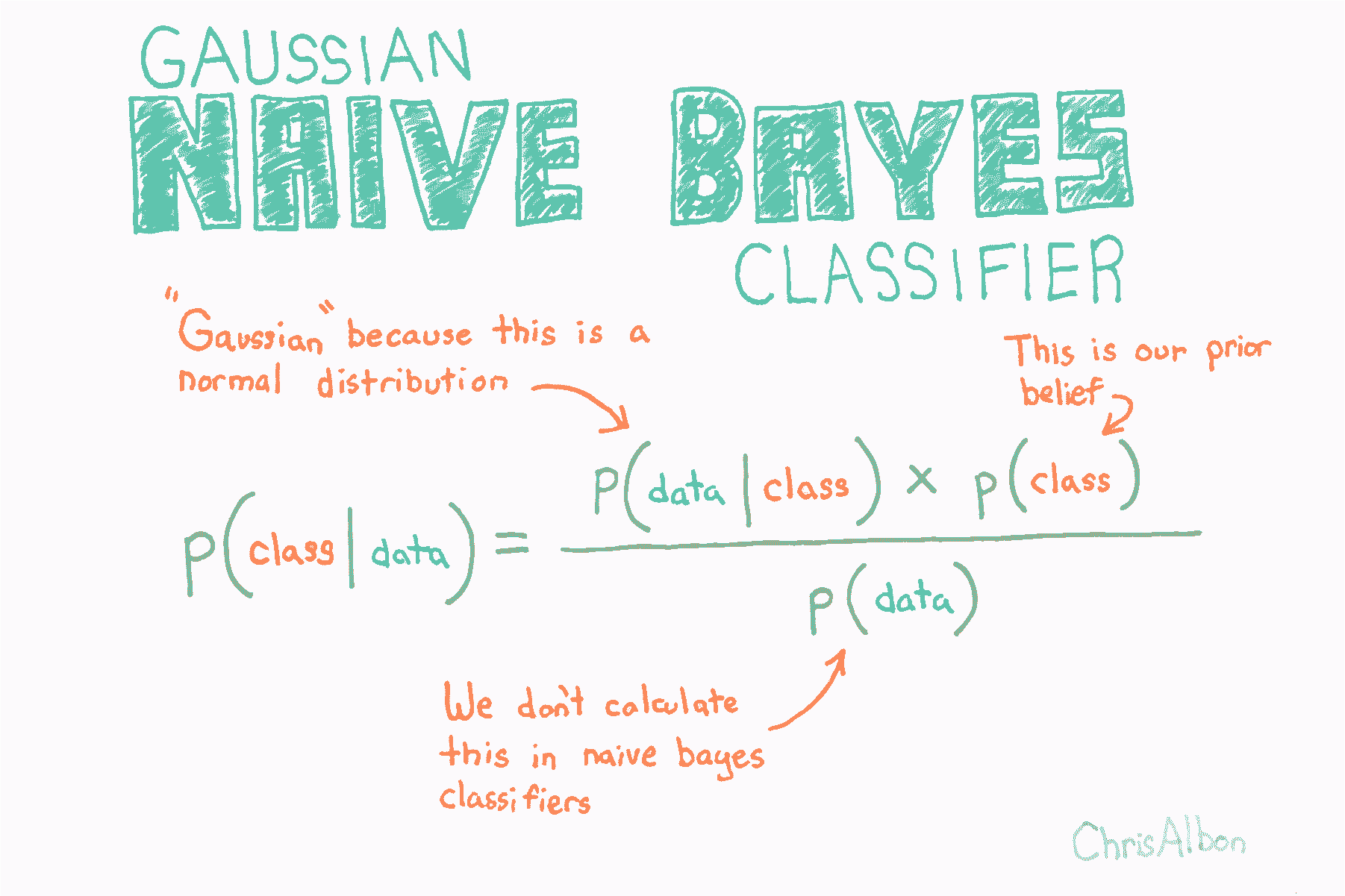``````# 加载库
from sklearn import datasets
from sklearn.naive_bayes import GaussianNB

# 加载数据
X = iris.data
y = iris.target

# 创建高斯朴素贝叶斯对象，带有每个类别的先验概率
clf = GaussianNB(priors=[0.25, 0.25, 0.5])

# 训练模型
model = clf.fit(X, y)

# 创建新的观测
new_observation = [[ 4,  4,  4,  0.4]]

# 预测类别
model.predict(new_observation)

# array()
``````

## 多项式逻辑回归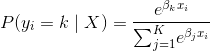``````# 加载库
from sklearn.linear_model import LogisticRegression
from sklearn import datasets
from sklearn.preprocessing import StandardScaler

# 加载数据
X = iris.data
y = iris.target

# 标准化特征
scaler = StandardScaler()
X_std = scaler.fit_transform(X)

# 创建 OVR 逻辑回归对象
clf = LogisticRegression(random_state=0, multi_class='multinomial', solver='newton-cg')

# 训练模型
model = clf.fit(X_std, y)

# 创建新的观测
new_observation = [[.5, .5, .5, .5]]

# 预测类别
model.predict(new_observation)

# array()

# 查看预测概率
model.predict_proba(new_observation)

# array([[ 0.01944996,  0.74469584,  0.2358542 ]])
``````

## 多项式朴素贝叶斯分类器

``````# 加载库
import numpy as np
from sklearn.naive_bayes import MultinomialNB
from sklearn.feature_extraction.text import CountVectorizer

# 创建文本
text_data = np.array(['I love Brazil. Brazil!',
'Brazil is best',
'Germany beats both'])

# 创建词袋
count = CountVectorizer()
bag_of_words = count.fit_transform(text_data)

# 创建特征矩阵
X = bag_of_words.toarray()

# 创建目标向量
y = np.array([0,0,1])

# 创建多项式朴素贝叶斯对象，带有每个类别的先验概率
clf = MultinomialNB(class_prior=[0.25, 0.5])

# 训练模型
model = clf.fit(X, y)

# 创建新的观测
new_observation = [[0, 0, 0, 1, 0, 1, 0]]

# 预测新观测的类别
model.predict(new_observation)

# array()
``````

## 从零编写朴素贝叶斯分类器

``````import pandas as pd
import numpy as np
``````

``````# 创建空数据帧
data = pd.DataFrame()

# 创建我们的目标变量
data['Gender'] = ['male','male','male','male','female','female','female','female']

# 创建我们的特征变量
data['Height'] = [6,5.92,5.58,5.92,5,5.5,5.42,5.75]
data['Weight'] = [180,190,170,165,100,150,130,150]
data['Foot_Size'] = [12,11,12,10,6,8,7,9]

# 查看数据
data
``````
Gender Height Weight Foot_Size
0 male 6.00 180 12
1 male 5.92 190 11
2 male 5.58 170 12
3 male 5.92 165 10
4 female 5.00 100 6
5 female 5.50 150 8
6 female 5.42 130 7
7 female 5.75 150 9

``````# 创建空数据帧
person = pd.DataFrame()

# 为这一行创建相同特征值
person['Height'] = 
person['Weight'] = 
person['Foot_Size'] = 

# 查看数据
person
``````
Height Weight Foot_Size
0 6 130 8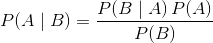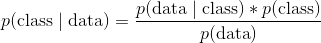•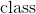是特定类别（例如男性）
•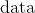是观测的数据
•称为后验
•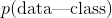叫做似然
•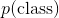叫做先验
•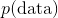叫做边缘概率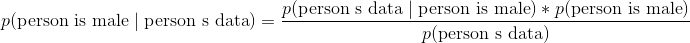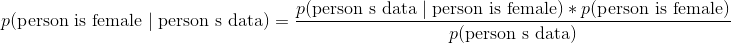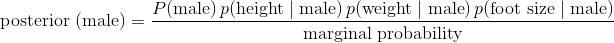•是先验概率。正如你所看到的，只是观测是男性的概率。 这只是数据集中的男性数量除以数据集中的总人数。
•是似然。注意我们已经解释了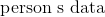所以它现在是数据集中的每个特征。“高斯”和“朴素”来自似然中的两个假设：
1. 如果你查看似然中的每项，你会注意到，我们假设每个特征彼此不相关。 也就是说，脚码与体重或身高等无关。这显然不是真的，而且是一个“朴素”的假设 - 因此称为“朴素贝叶斯”。
2. 其次，我们假设特征的值（例如女性的身体，女性的体重）通常是高斯分布的。这意味着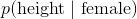是通过将所需参数输入正态分布的概率密度函数来计算的：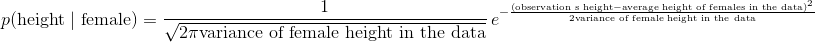•可能是贝叶斯方法中最令人困惑的部分之一。 在玩具示例（包括我们的）中，完全可以计算边际概率。 但是，在许多实际情况中，要找到边际概率的值极其困难或不可能（解释为什么超出了本教程的范围）。 对于我们的分类器来说，这并不像你想象的那么严重。 为什么？ 因为我们不关心真正的后验值是什么，我们只关心哪个类具有最高的后验值。 并且因为边际概率对于所有类别都是相同的，（1）我们可以忽略分母，（2）只计算每个类的后验分子，（3）选择最大的分子。 也就是说，我们可以忽略后验分母，并仅根据后验分子的相对值进行预测。

``````# 男性数量
n_male = data['Gender'][data['Gender'] == 'male'].count()

# 女性数量
n_female = data['Gender'][data['Gender'] == 'female'].count()

# 总行数
total_ppl = data['Gender'].count()

# 男性比例
P_male = n_male/total_ppl

# 女性比例
P_female = n_female/total_ppl
````````````# 按性别分组数据，并计算每个特征的均值
data_means = data.groupby('Gender').mean()

# 查看值
data_means
``````
Height Weight Foot_Size
Gender
female 5.4175 132.50 7.50
male 5.8550 176.25 11.25
``````# 按性别分组数据，并计算每个特征的方差
data_variance = data.groupby('Gender').var()

# 查看值
data_variance
``````
Height Weight Foot_Size
Gender
female 0.097225 558.333333 1.666667
male 0.035033 122.916667 0.916667

``````# 男性的均值
male_height_mean = data_means['Height'][data_variance.index == 'male'].values
male_weight_mean = data_means['Weight'][data_variance.index == 'male'].values
male_footsize_mean = data_means['Foot_Size'][data_variance.index == 'male'].values

# 男性的方差
male_height_variance = data_variance['Height'][data_variance.index == 'male'].values
male_weight_variance = data_variance['Weight'][data_variance.index == 'male'].values
male_footsize_variance = data_variance['Foot_Size'][data_variance.index == 'male'].values

# Means for female
female_height_mean = data_means['Height'][data_variance.index == 'female'].values
female_weight_mean = data_means['Weight'][data_variance.index == 'female'].values
female_footsize_mean = data_means['Foot_Size'][data_variance.index == 'female'].values

# Variance for female
female_height_variance = data_variance['Height'][data_variance.index == 'female'].values
female_weight_variance = data_variance['Weight'][data_variance.index == 'female'].values
female_footsize_variance = data_variance['Foot_Size'][data_variance.index == 'female'].values
``````

``````# 创建计算 p(x | y) 的函数
def p_x_given_y(x, mean_y, variance_y):

# 将参数输入到概率密度函数
p = 1/(np.sqrt(2*np.pi*variance_y)) * np.exp((-(x-mean_y)**2)/(2*variance_y))

# 返回 p
return p
``````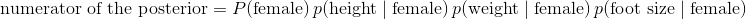``````# 如果未分类的观测是男性的后验分子
P_male * \
p_x_given_y(person['Height'], male_height_mean, male_height_variance) * \
p_x_given_y(person['Weight'], male_weight_mean, male_weight_variance) * \
p_x_given_y(person['Foot_Size'], male_footsize_mean, male_footsize_variance)

# 6.1970718438780782e-09
``````
``````# 如果未分类的观测是女性的后验分子
P_female * \
p_x_given_y(person['Height'], female_height_mean, female_height_variance) * \
p_x_given_y(person['Weight'], female_weight_mean, female_weight_variance) * \
p_x_given_y(person['Foot_Size'], female_footsize_mean, female_footsize_variance)

# 0.00053779091836300176
``````